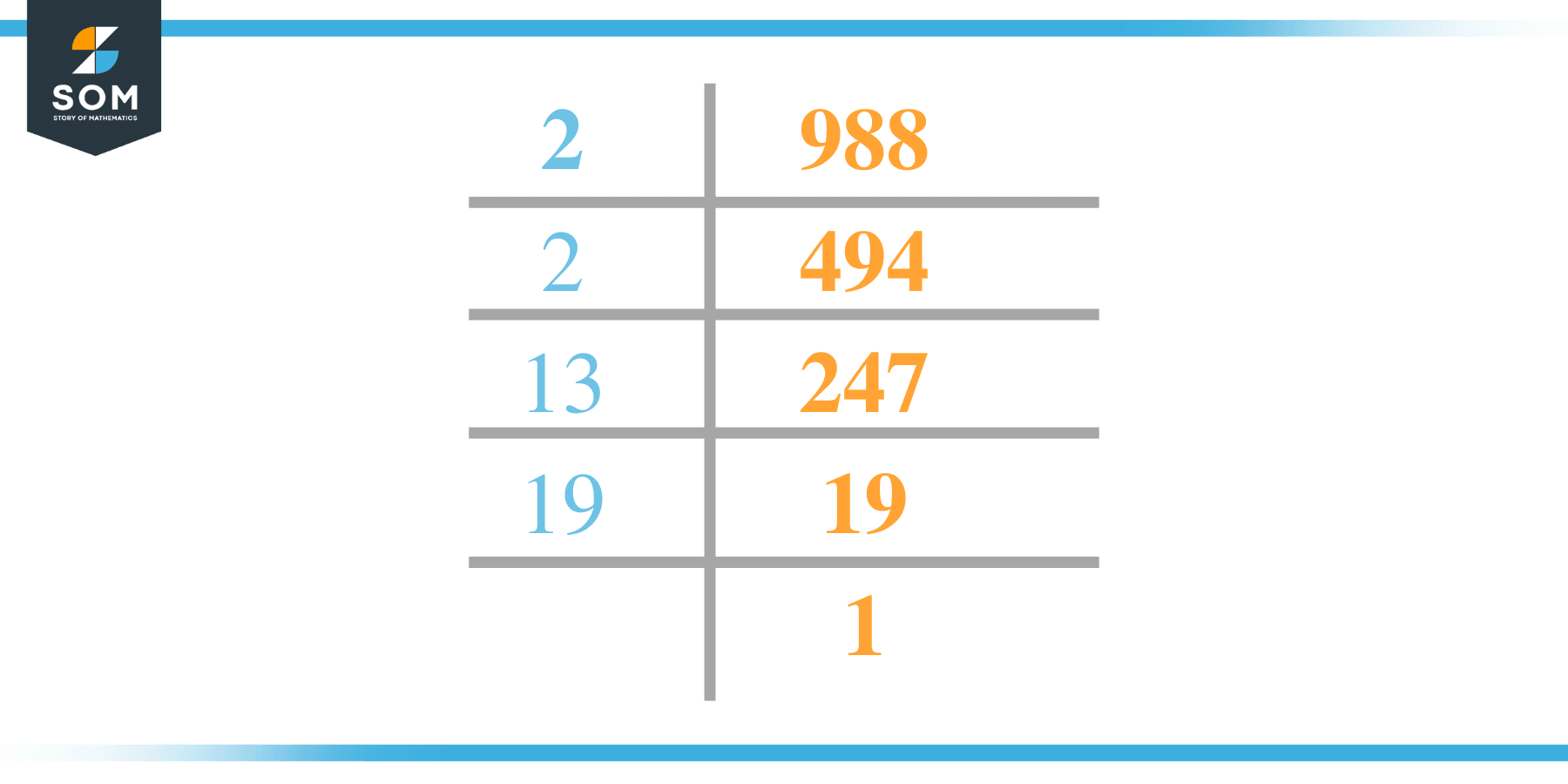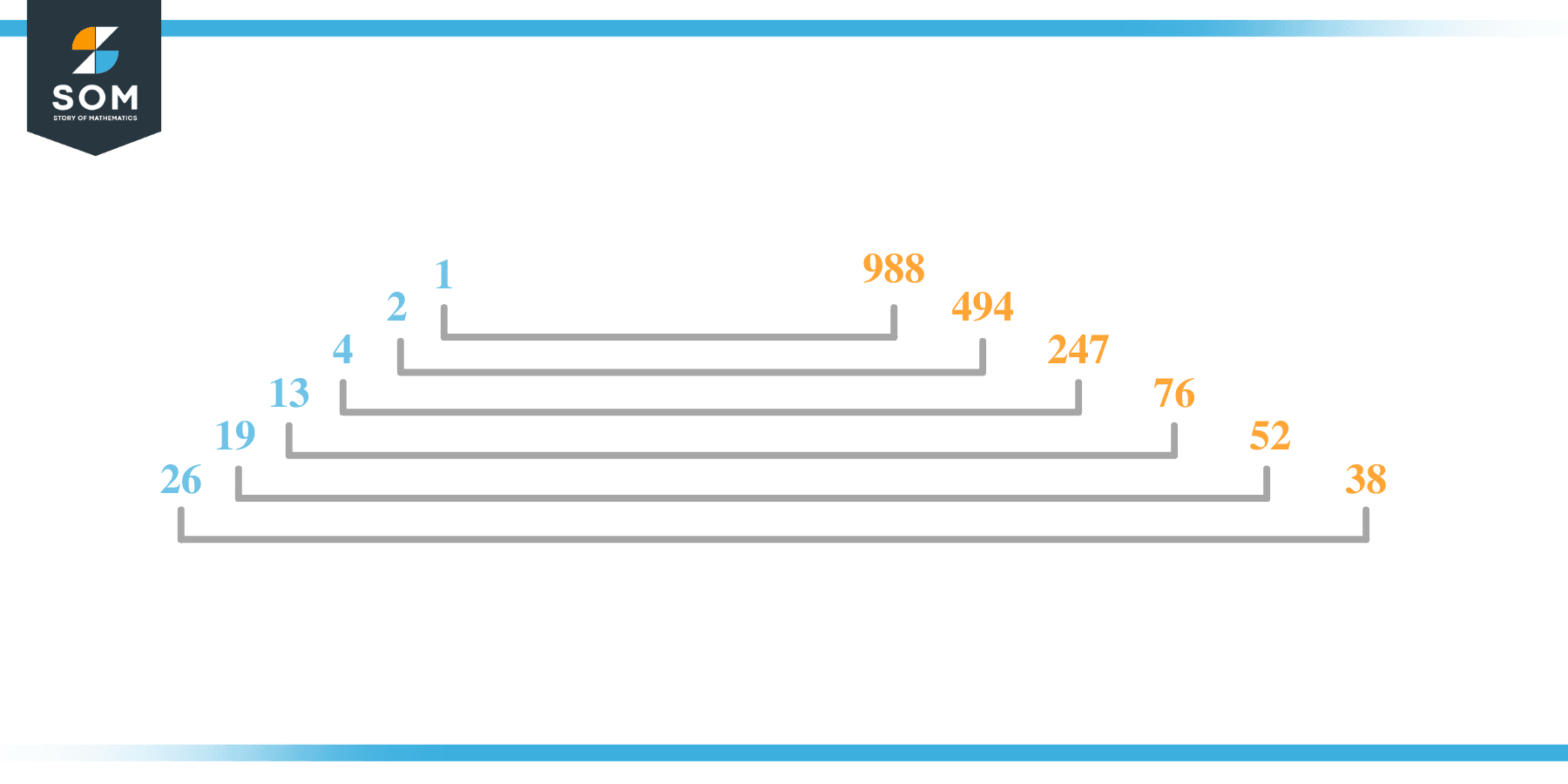# Factors of 988: Prime Factorization, Methods, and Examples

988 is said to be an even number as well as a composite number because it includes more than 2 factors.The number 988 has 12 positive factors which can be used as divisors with 988 as a dividend their division result in 12 whole numbers without any decimal places.

### Factors of 988

Here are the factors of number 988.

Factors of 988: 1, 2, 4, 13, 19, 26, 38, 52, 76, 247, 494 and 988

### Negative Factors of 988

The negative factors of 988 are similar to its positive aspects, just with a negative sign.

Negative Factors of 988: -1, -2, -4, -13, -19, -26, -38, -52, -76, -247, -494 and -988

### Prime Factorization of 988

The prime factorization of 988 is the way of expressing its prime factors in the product form.

Prime Factorization: 2 x 2 x 13 x 19

In this article, we will learn about the factors of 988 and how to find them using various techniques such as upside-down division, prime factorization, and factor tree.

## What Are the Factors of 988?

The factors of 988 are 1, 2, 4, 13, 19, 26, 38, 52, 76, 247, 494 and 988. These numbers are the factors as they do not leave any remainder when divided by 988.

The factors of 988 are classified as prime numbers and composite numbers. The prime factors of the number 988 can be determined using the prime factorization technique.

## How To Find the Factors of 988?

You can find the factors of 988 by using the rules of divisibility. The divisibility rule states that any number, when divided by any other natural number, is said to be divisible by the number if the quotient is the whole number and the resulting remainder is zero.

To find the factors of 988, create a list containing the numbers that are exactly divisible by 988 with zero remainders. One important thing to note is that 1 and 988 are the 988’s factors as every natural number has 1 and the number itself as its factor.

1 is also called the universal factor of every number. The factors of 988 are determined as follows:

$\dfrac{988}{1} = 988$

$\dfrac{988}{2} = 494$

$\dfrac{988}{4} = 247$

$\dfrac{988}{13} = 76$

$\dfrac{988}{19} = 52$

$\dfrac{988}{26} = 38$

$\dfrac{988}{38} = 26$

$\dfrac{988}{52} = 19$

$\dfrac{988}{76} = 13$

$\dfrac{988}{247} = 4$

$\dfrac{988}{494} = 2$

$\dfrac{988}{988} = 1$

Therefore, 1, 2, 4, 13, 19, 26, 38, 52, 76, 247, 494 and 988 are the factors of 988.

### Total Number of Factors of 988

For 988, there are 12 positive factors and 12 negative ones. So in total, there are 24 factors of 988.

To find the total number of factors of the given number, follow the procedure mentioned below:

1. Find the factorization/prime factorization of the given number.
2. Demonstrate the prime factorization of the number in the form of exponent form.
3. Add 1 to each of the exponents of the prime factor.
4. Now, multiply the resulting exponents together. This obtained product is equivalent to the total number of factors of the given number.

By following this procedure, the total number of factors of 988 is given as:

Factorization of 988 is 2 x 2 x 13 x 19.

The exponent of 2, 13, and 19 is 2,1,1.

Adding 1 to each and multiplying them together results in 12.

Therefore, the total number of factors of 988 is 24. Twelve are positive, and twelve factors are negative.

### Important Notes

Here are some essential points that must be considered while finding the factors of any given number:

• The factor of any given number must be a whole number.
• The factors of the number cannot be in the form of decimals or fractions.
• Factors can be positive as well as negative.
• Negative factors are the additive inverse of the positive factors of a given number.
• The factor of a number cannot be greater than that number.
• Every even number has 2 as its prime factor, the smallest prime factor.

## Factors of 988 by Prime FactorizationThe number 988 is a composite. Prime factorization is a valuable technique for finding the number’s prime factors and expressing the number as the product of its prime factors.

Before finding the factors of 988 using prime factorization, let us find out what prime factors are. Prime factors are the factors of any given number that are only divisible by 1 and themselves.

To start the prime factorization of 988, start dividing by its most minor prime factor. First, determine that the given number is either even or odd. If it is an even number, then 2 will be the smallest prime factor.

Continue splitting the quotient obtained until 1 is received as the quotient. The prime factorization of 988 can be expressed as:

988 = 2 x 2 x 13 x 19

## Factors of 988 in PairsThe factor pairs are the duplet of numbers that, when multiplied together, result in the factorized number. Factor pairs can be more than one depending on the total number of factors given.

For 988, the factor pairs can be found as:

1 x 988 = 988

2 x 494 = 988

4 x 247 = 988

13 x 76 = 988

19 x 52 = 988

26 x 38 = 988

The possible factor pairs of 988 are given as (1, 988), (2, 494), (4, 247), (13, 76), (19, 52) and (26, 38).

All these numbers in pairs, when multiplied, give 988 as the product.

The negative factor pairs of 988 are given as:

-1 x -988 = 988

-2 x -494 = 988

-4 x -247 = 988

-13 x -76 = 988

-19 x -52 = 988

-26 x -38 = 988

It is important to note that in negative factor pairs, the minus sign has been multiplied by the minus sign, due to which the resulting product is the original positive number. Therefore, -1, -2, -4, -13, -19, -26, -38, -52, -76, -247, -494 and -988 are called negative factors of 988.

The list of all the factors of 988, including positive as well as negative numbers, is given below.

Factor list of 988: 1,-1, 2, -2, 4, -4, 13, -13, 19, -19, 26, -26, 38, -38, 52, -52, 76, -76, 247, -247, 494, -494, 988, and -988

## Factors of 988 Solved Examples

To better understand the concept of factors, let’s solve some examples.

### Example 1

How many factors of 988 are there?

### Solution

The total number of Factors of 988 is 12.

Factors of 988 are 1, 2, 4, 13, 19, 26, 38, 52, 76, 247, 494 and 988.

### Example 2

Find the factors of 988 using prime factorization.

### Solution

The prime factorization of 988 is given as:

988 $\div$ 2 = 494

494 $\div$ 2 = 247

247 $\div$ 13 = 19

19 $\div$ 19 = 1

So the prime factorization of 988 can be written as:

2 x 2 x 13 x 19 = 988